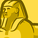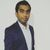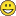# QlikView App Dev

Discussion Board for collaboration related to QlikView App Development.

cancel
Showing results for
Did you mean:Creator II

## Sum of Rows in a Text Box

How do I get Sum of Rows value on a Expression/Field from Straight Table in a Text Box?

1 Solution

Accepted SolutionsCreator II
Author

The Expression is a simple Count(Field) but there are many dimensions in the Straight Table. Should I list all the dimensions under Aggr() ? I don't show all the Dimensions at once. What happens then.

6 RepliesMVP

It depends on what dimensions and expressions you are using in your straight table.

Can you provide dimensions and expressions here?Contributor III

QW,

You could use the aggr() function inside a sum() to get the same result as a sum of rows in a table.  The aggr() would calculate the expression for each combination of dimension values - just like straight table does.

I hope that helps.Creator II
Author

The Expression is a simple Count(Field) but there are many dimensions in the Straight Table. Should I list all the dimensions under Aggr() ? I don't show all the Dimensions at once. What happens then.MVP

You can use something like this

SUM(Aggr(Count(Field),Dim1,Dim2,Dim3))MVPStrange